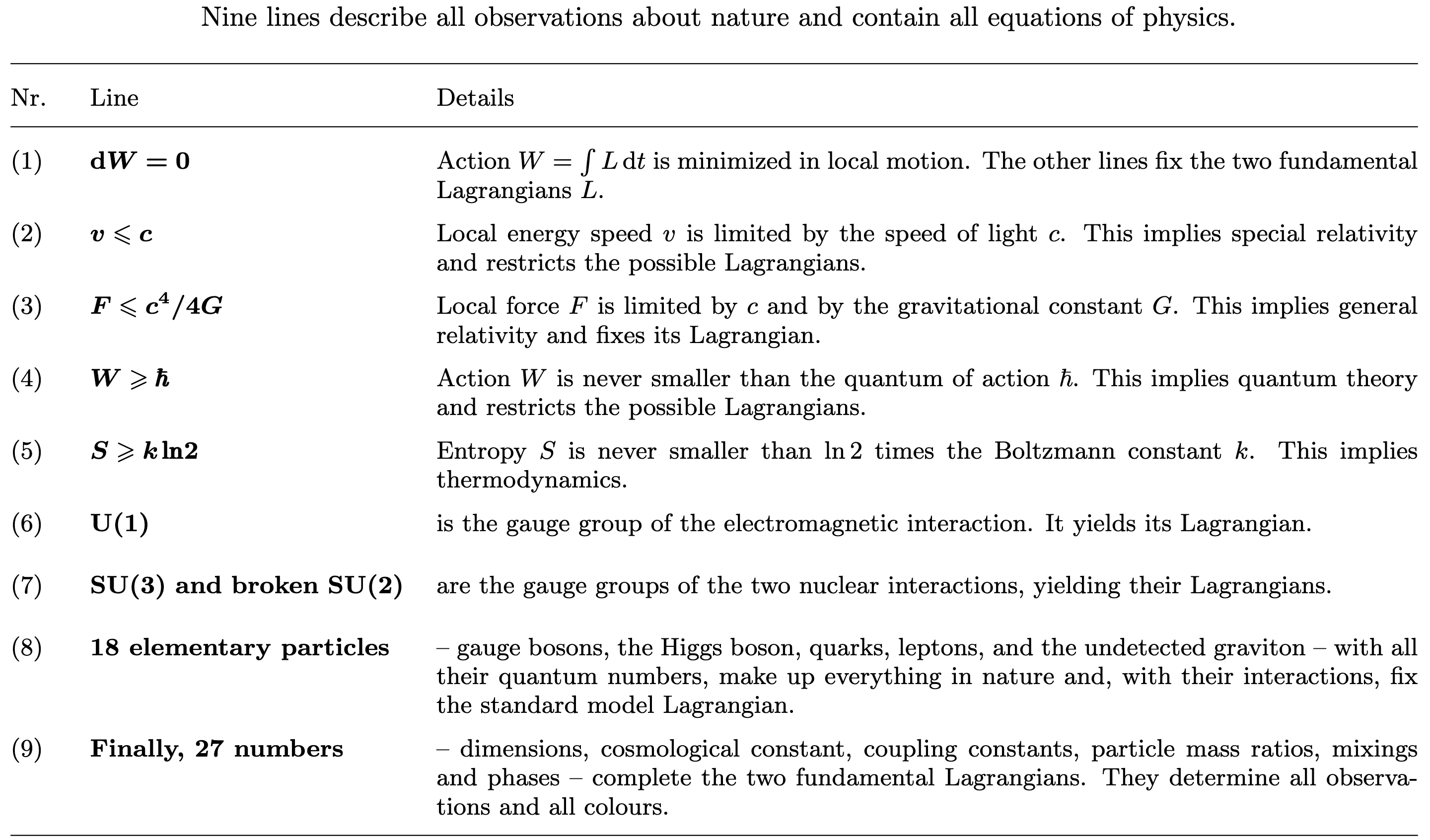The present page is written to make the reader wonder, check, and be amazed.
Everything here has been thoroughly checked by thousands of scientists. (See here on how this is done.)

### This 9-line summary of textbook physics agrees with 400 years of experiments. Exactly.

The 9 lines, typeset in bold, state that general relativity, the standard model of particle physics and thermodynamics describe all of nature.

The 9 lines contain everything we know about fundamental physics and everything we do not.

*

The 9 lines contain all present knowledge about nature, including all textbook physics and all observations ever made.

The simplicity of the 9 lines and their vast domain of validity are intensely fascinating.

No known observation and no known measurement contradicts these 9 lines, not even in the last significant digit.

Find a single contradicting observation or experiment and become famous.

The 9 lines determine all equations of physics:

Lines 1, 2 and 3, together, uniquely determine special and general relativity, cosmology, the Hilbert Lagrangian and Einstein's field equations, as told here.

Line 5 uniquely implies thermodynamics. The references are given in the pdf linked above.

Lines 1, 2, 4 and 6 to 9, together, uniquely determine quantum field theory and the Lagrangian of the standard model of particle physics, with massive neutrinos and PMNS mixing – often written with a Lagrangian taking a full page in a small font.

The 9 lines show how little math is required to describe nature with high precision.

The 9 lines resulted from the work of countless scientists and engineers during 400 years.

Galileo started around the year 1600; line 1 resulted after over 150 years. Line 5 arose from 1824 to 1929, line 6 arose
around 1860, lines 2 and 4 around 1900, line 3 from 1915 to 2002, and lines 7, 8 and 9 from 1936 to 1973.

The 9 lines contain all natural sciences.

They contain physics, chemistry, material science, biology, medicine, geology, astronomy and engineering.

Again: any single observation contradicting the 9 lines will create a sensation.

*

Three questions are open in fundamental physics.

III    Are all observations in nature described by the 9 lines – or are there phenomena beyond present textbook physics?

For example, to which line do dark matter and dark energy belong exactly?c4

II    Are there any undiscovered lines with new physics – or will  F ≤ c4/4G  remain the last law of physics?

Explored mainly in the years from 1973 to 2002, the force limit is the law of physics discovered last.4
The limit value c4/4G ≈ 3.0 · 1043 N for the local force has passed all checks.4
It allows deducing Einstein's field equations and is the shortest known summary of general relativity.4
Because line 3 on maximum force c4/4G was added to the list only recently, a separate web page is dedicated to it.c4

IIII    What is the common explanation for the 9 lines?c4

What determines the principles in lines 1 to 5?c4
Lines 1 to 5 start from continuous (curved) space and time but imply that there is a smallest length and time interval. How can this be? c4
What determines the choices in lines 6 to 9, i.e., the force spectrum, the particle spectrum and the fundamental constants?c4
The last four lines contain the only known physics effects unexplained by and thus beyond the standard model. Why?c4

Many enjoy searching for answers.

*

This image about how to summarize physics and all natural sciences in 9 lines might be useful and is free for reuse.*

A video summary, by David Tong, of the standard model and of general relativity is found at https://www.youtube.com/watch?v=Unl1jXFnzgo&t=1s.
The mathematical expression in the video can be seen as a symbolic combination of the 9 lines. In contrast, the 9 lines are complete and precise.

Line 1 is part of David Tong's mentioned video and also the topic of Sabine Hossenfelder's video on least action at https://www.youtube.com/watch?v=A0da8TEeaeE.

Are the 9 lines a "theory of everything"? They do fulfill all requirements found in tabloids and in popular books. In fact, they do so since decades.
But the 9 lines are not a "theory of everything": the origin of lines 6 to 9 has to be clarified first. (An attempt is found here.)

*

The 9 lines can be combined. Together, they imply that twice the Planck length (4G h-bar/c^3)^(1/2) is the smallest length in nature. This is not often told.

Conversely, the assumption of continuous space implies a vanishing Planck length. This implies a vanishing quantum of action, or a vanishing gravitational constant, or both. This also implies the lack of most physical measurement units.

In other words, if continuity is taken as an exact property of nature, then it contradicts all of modern physics.

It may be comforting to note that this and all similar issues involving gravity and quantum theory disappear if space is seen as (effectively) continuous for all lengths longer than twice the Planck length - as implied by relativistic quantum gravity.

Therefore, the 9 lines imply that continuous space is an approximation. Continuity is valid for all practical purposes, and in particular, for lengths longer than twice the Planck length, the smallest length in nature. Details in Researchgate at https://www.researchgate.net/publication/359705442.

*    *